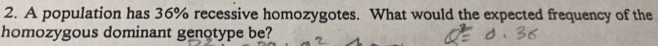# Problem: A population has 36% recessive homozygotes. What would the expected frequency of the homozygous dominant genotype be?

###### FREE Expert Solution

Since no other details were mentioned, this can only be solved if we assume that it follows the Hardy-Weinberg model. The frequencies of alleles follow the equation:

p + q = 1, where###### Problem Details

A population has 36% recessive homozygotes. What would the expected frequency of the homozygous dominant genotype be?